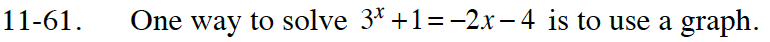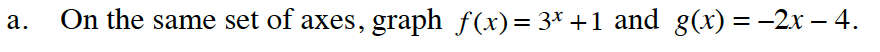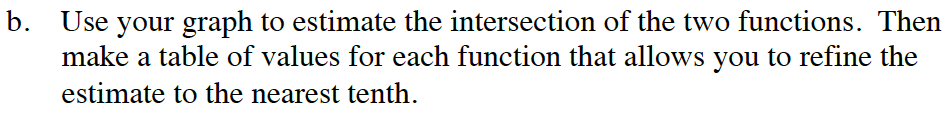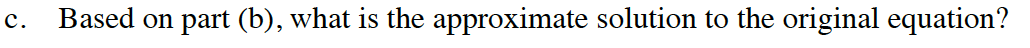Home > CC4 > Chapter 11 > Lesson 11.2.2 > Problem11-61

11-61.
1. One way to solve 3x + 1 = −2x − 4 is to use a graph. 11-61 HW eTool (Desmos). Homework Help ✎

1. On the same set of axes, graph f(x) = 3x + 1 and g(x) = −2x − 4.

2. Use your graph to estimate the intersection of the two functions. Then make a table of values for each function that allows you to refine the estimate to the nearest tenth.

3. Based on part (b), what is the approximate solution to the original equation?Use the eTool below to graph the equations.
Click the link at the right to view the full version of the eTool: Int1 11-61 HW eTool.